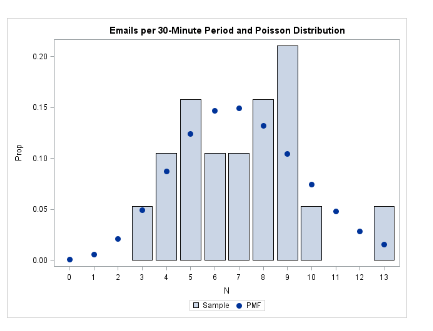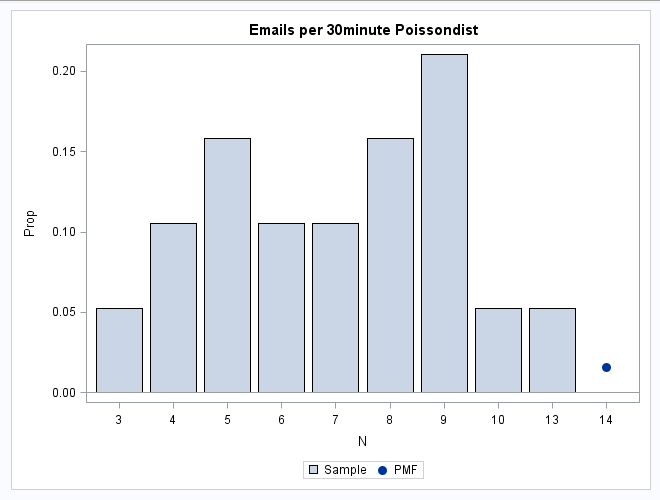## overlay fitted density Poisson data

Dear SAS Help,

I use SAS 9.4

I tried the exercise on this link: https://blogs.sas.com/content/iml/2012/04/04/fitting-a-poisson-distribution-to-data-in-sas.html

This is the code I copied from the site.

--------------------------------------

``````data MyData;
input N @@;
datalines;
7 7 13 9 8 8 9 9 5 6 6 9 5 10 4 5 3 8 4
;
run;

proc freq data=MyData;
tables N / out=FreqOut plots=FreqPlot (scale=percent);
run;

proc genmod data=MyData;
model N= /dist=poisson;
output out= PoissonFit p=lambda;
run;

data _null_;
set Poissonfit;
call symputx("Lambda", Lambda);
stop;
run;

data PMF;
do t= 0 to 13;
Y = pdf("Poisson", t, &Lambda);
end;
run;

data Discrete;
merge FreqOut PMF;
Prop = Percent / 100;
run;

*\bij dit stukje is er een probleem. Ik heb gemaild naar de site op 15 januari;
proc sgplot data=Discrete;
vbarparm category=N response=Prop /legendlabel = 'Sample';
scatter x=T y=Y / legendlabel= 'PMF'
title "Emails per 30minute Poissondist";
run;

``````

-----------------------------------------------------------------------

The log gives this error message:

NOTE: Since no format is assigned, the numeric category variable will use the default of BEST6.

The plot that I should get should look like the upper plot, but my result is the lower plot.

Questions:

1. What did I do wrong?

2. Is it possible to have a line instead of dots.

3. Can I use these steps to do an overlay on a poisson density plot created with the FMM procedure?The plot I get looks like this:4 REPLIES 4

## Re: overlay fitted density Poisson data

Hi

The dataset PMF probably doesn't contain what you expect.

Try adding the word output as below.  Then you should get the observations you expect.

data PMF; do t= 0 to 13;
Y = pdf("Poisson", t, &Lambda); output;
end;
run;

To get a line instead of circles, try changing the word scatter to series.

Re question 3: sorry, I don't know.

Hope that helps.

## Re: overlay fitted density Poisson data

1) You need an OUTPUT Statement in your do look. As of now, you are creating just 1 observation in your PMD data set

``````data PMF;
do t= 0 to 13;
Y = pdf("Poisson", t, &Lambda);
output;
end;
run;``````

2) Yes, it is possible, simply change the SCATTER Statement to a SERIES Statement like this. Use the MARKERATTRS Option to control the markers and delete both the MARKER and MARKERATTRS Options

``````proc sgplot data=Discrete;
vbarparm category=N response=Prop /legendlabel = 'Sample';
series x=T y=Y / markers legendlabel= 'PMF'
title "Emails per 30minute Poissondist";
run;``````

3) Show us your PROC FMM code 🙂 You can always extract underlying data from procedure output with an ODS OUTPUT statement.

## Re: overlay fitted density Poisson data

Thank you very much. This worked.

Here is my proc fmm code. The data file is attached.

``````proc fmm data=acu plots=density (bins=15);
model frequency= /dist=poisson  k=3
parms(2.1, 2.8, 3.2);  *\starting values for mean ln(8), ln(16) en ln(24);
probmodel / parms (0, 0);
freq age;
title 'poisson with 3 support points';
run;``````

## Re: overlay fitted density Poisson data

Do something like this and change the Component value in the Where Statement to get another Component model 🙂

``````proc fmm data=acu plots=density (bins=15);
model frequency= /dist=poisson  k=3
parms(2.1, 2.8, 3.2);  *\starting values for mean ln(8), ln(16) en ln(24);
probmodel / parms (0, 0);
freq age;
title 'poisson with 3 support points';

ods output ParameterEstimates=pePoisson;
run;

data _null_;
set pePoisson;
where Component=1;
call symputx('lambdaFMM', Estimate);
run;

%put &lambdaFMM.;

data PMF_fmm;
do t= 0 to 13;
Y = pdf("Poisson", t, &lambdaFMM.);
output;
end;
run;

data Discrete_fmm;
merge FreqOut PMF_fmm;
Prop = Percent / 100;
run;

proc sgplot data=Discrete_fmm;
vbarparm category=N response=Prop /legendlabel = 'Sample';
series x=T y=Y / markers legendlabel= 'PMF'